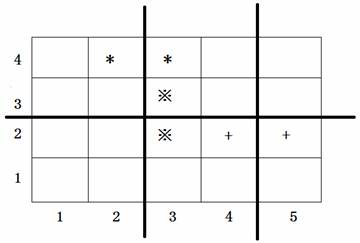# P1056 排座椅

• 14.3K通过
• 41.6K提交
• 题目提供者 CCF_NOI
• 评测方式 云端评测
• 标签 排序 贪心 NOIp普及组 2008
• 难度 普及/提高-
• 时空限制 1000ms / 128MB
• 提示：收藏到任务计划后，可在首页查看。

## 题目描述

上课的时候总会有一些同学和前后左右的人交头接耳，这是令小学班主任十分头疼的一件事情。不过，班主任小雪发现了一些有趣的现象，当同学们的座次确定下来之后，只有有限的D对同学上课时会交头接耳。

同学们在教室中坐成了$M$行$N$列，坐在第i行第j列的同学的位置是$(i,j)$，为了方便同学们进出，在教室中设置了$K$条横向的通道，$L$条纵向的通道。

于是，聪明的小雪想到了一个办法，或许可以减少上课时学生交头接耳的问题：她打算重新摆放桌椅，改变同学们桌椅间通道的位置，因为如果一条通道隔开了$2$个会交头接耳的同学，那么他们就不会交头接耳了。

请你帮忙给小雪编写一个程序，给出最好的通道划分方案。在该方案下，上课时交头接耳的学生的对数最少。

## 输入输出格式

输入格式：

第一行，有$5$个用空格隔开的整数，分别是$M,N,K,L,D(2 \le N,M \le 1000,0 \le K<M,0 \le L<N,D \le 2000)$

接下来的$D$行，每行有$4$个用空格隔开的整数。第$i$行的$4$个整数$X_i,Y_i,P_i,Q_i$，表示坐在位置$(X_i,Y_i)$与$(P_i,Q_i)$的两个同学会交头接耳（输入保证他们前后相邻或者左右相邻）。

输入数据保证最优方案的唯一性。

输出格式：

共两行。
第一行包含$K$个整数$a_1,a_2,…,a_K$，表示第$a_1$行和$a_1+1$行之间、第$a-2$行和$a_2+1$行之间、…、第$a_K$行和第$a_K+1$行之间要开辟通道，其中$a_i< a_i+1$，每两个整数之间用空格隔开（行尾没有空格）。

第二行包含$L$个整数$b_1,b_2,…,b_L$，表示第$b_1$列和$b_1+1$列之间、第$b_2$列和$b_2+1$列之间、…、第$b_L$列和第$b_L+1$列之间要开辟通道，其中$b_i< b_i+1$，每两个整数之间用空格隔开（列尾没有空格）。

## 输入输出样例

输入样例#1： 复制
4 5 1 2 3
4 2 4 3
2 3 3 3
2 5 2 4

输出样例#1： 复制
2
2 4


## 说明上图中用符号*、※、+标出了$3$对会交头接耳的学生的位置，图中$3$条粗线的位置表示通道，图示的通道划分方案是唯一的最佳方案。

2008年普及组第二题

提示
标程仅供做题后或实在无思路时参考。
请自觉、自律地使用该功能并请对自己的学习负责。
如果发现恶意抄袭标程，将按照I类违反进行处理。